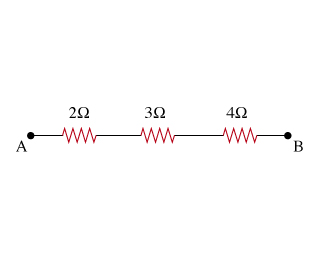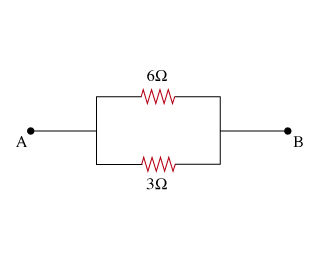# Problem: 1. For the combination of resistors shown, find the equivalent resistance between points A and B.Express your answer in Ohms.2. If the circuit is, as shown below find the equivalent resistance between points Aand B.

###### FREE Expert Solution

1.

The resistors in the figure are in series.

Equivalent resistance for resistors in series is given by:

$\overline{){{\mathbf{R}}}_{{\mathbf{eq}}}{\mathbf{=}}{{\mathbf{R}}}_{{\mathbf{1}}}{\mathbf{+}}{{\mathbf{R}}}_{{\mathbf{2}}}{\mathbf{+}}{\mathbf{.}}{\mathbf{.}}{\mathbf{.}}{\mathbf{+}}{{\mathbf{R}}}_{{\mathbf{n}}}}$

80% (436 ratings)###### Problem Details

1. For the combination of resistors shown, find the equivalent resistance between points A and B.